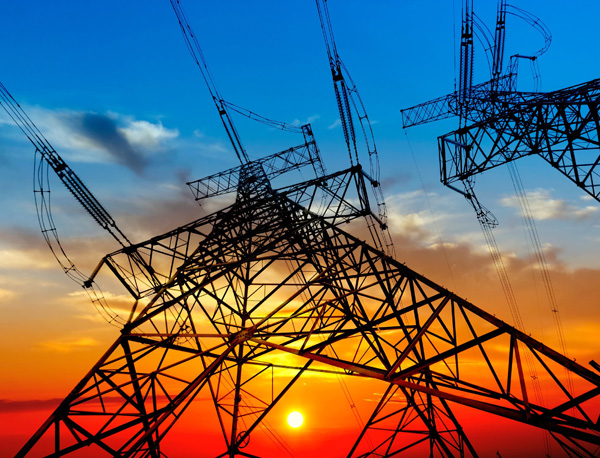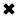# Electrical Energy Definition Explained

#### By R. W. Hurst, Editor#### Electrical Energy is defined as the ability to do work

Electrical Energy is energy stored in an electric field or transported by an electric current. Energy is defined as the ability to do work, and electrical energy is simply one of the many types of energy. Examples of electrical energy include:

• the Electrical Energy that is constantly stored in the Earth's atmosphere, and is partly released during a thunderstorm in the form of lightning
• the energy that is stored in the coils of an electrical generator in a power plant (which is generating electrical energy), and is then transmitted by wires to the consumer; the consumer then pays for each unit of a form of electrical energy received
• the forms of energy that is stored in a capacitor, and can be released to drive a current through an electrical circuit

Electric charge

An Electric charge is a property of certain subatomic particles (e.g., electrons and protons) which interacts with electro magnetic field and causes attractive and repulsive forces between them. Electric charges give rise to one of the four fundamental forces of nature, and is a conserved property of matter that can be quantified. In this sense, the phrase "quantity of electricity" is used interchangeably with the phrases "charge of electricity" and "quantity of charge." There are two types of charge: we call one kind of charge positive and the other negative. Through experimentation, we find that like-charged objects repel and opposite-charged objects attract one another. The magnitude of the force of attraction or repulsion is given by Coulomb's law.

Electric field

The concept of Electrical Energy field was introduced by Michael Faraday. The electrical field force acts between two charges, in the same way that the gravitational field force acts between two masses. However, the electric field is a little bit different. Gravitational force depends on the masses of two bodies, whereas electric force depends on the electric charges of two bodies. While gravity can only pull two masses together, the electric force can be an attractive or repulsive force. If both charges are of same sign (e.g. both positive), there will be a repulsive force between the two. If the charges are opposite, there will be an attractive force between the two bodies (we see this in static electricity and kinetic energy). The magnitude of the force varies inversely with the square of the distance between the two bodies, and is also proportional to the product of the unsigned magnitudes of the two charges.

Electric potential energy

The electric potential difference between two points is defined as the work done per unit charge (against electrical forces) in moving a positive point charge slowly between two points. If one of the points is taken to be a reference point with zero potential, then the electric potential at any point can be defined in terms of the work done per unit charge in moving a positive point charge from that reference point to the point at which the potential is to be determined. For isolated charges, the reference point is usually taken to be infinity. The potential is measured in volts. (1 volt = 1 joule/coulomb) The electric potential is analogous to temperature: there is a different temperature at every point in space, and the temperature gradient indicates the direction and magnitude of the driving force behind heat flow energy source. Similarly, there is an electric potential at every point in space, and its gradient indicates the direction and magnitude of the driving force behind moving charged energy.

Electric current

An Electrical Energy current is a flow of electric charge, and its intensity is measured in amperes. Examples of electric currents include metallic conduction, where electrons flow through a conductor such as a metal wire, and electrolysis, where ions (charged atoms) flow through liquids. The particles themselves often have energy to move quite slowly, while the moving electric field that drives them propagates at close to the speed of light. See electrical conduction for more information.

Devices that use charge flow principles in materials are called electronic devices.

A direct current (DC) is a unidirectional flow, while an alternating current (AC) reverses direction repeatedly. The time average of an alternating current is zero, but its energy capability (RMS value) is not zero.

Ohm's Law is an important relationship describing the behaviour of electric currents, relating them to voltage.

For historical reasons, Electrical Energy current is said to flow from the most positive part of a circuit to the most negative part. The electric current thus defined is called conventional current. It is now known that, depending on the conditions, an electric current can consist of a flow of charged particles in either direction, or even in both directions at once. The positive-to-negative convention is widely used to simplify this situation. If another definition is used - for example, "electron current" - it should be explicitly stated.

##### Basic Electricity Handbook, Vol. 1• FULLY ILLUSTRATED
• GREAT FOR SCHOOL PROJECTS
• GREAT PRICE: \$5.99
• ...

This 100+ page e-book is a great guide for those who have a basic interest in the field of electricity. This well-illustrated e-book, coupled with some basic knowledge of electricity, will give you a broad theoretical background in this fundamental subject.

CONTENTS
• FUNDAMENTALS OF ELECTRICITY
• HOW DOES ELECTRICITY WORK?
• HOW IS ELECTRICITY GENERATED?
• WHERE DOES ELECTRICITY COME FROM?
• BASIC HOME WIRING
• ELECTRICITY FOR STUDENTS
• ENERGY SAVING TIPS

##### TRAINING EF COURSES

Content Community Connection
Top